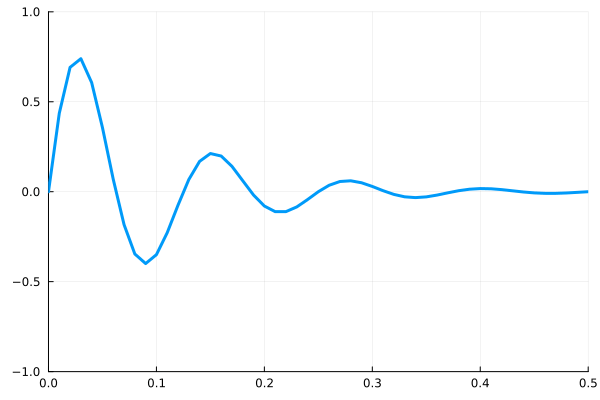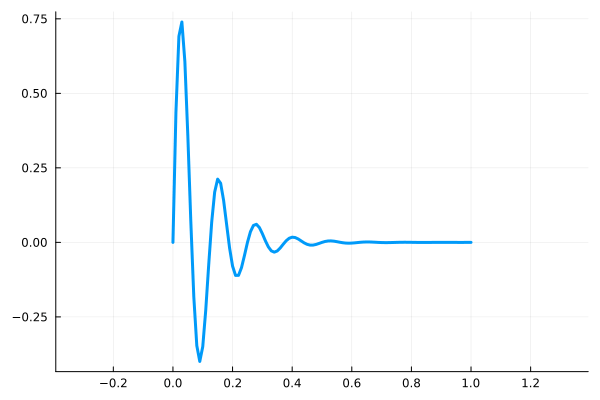## Axis limits

You can control where data appears in the axes by setting xlimits and ylimits.

using Plots
x = 0:0.01:1;
y = @. sin(8*2π*x)/exp(10*x);

plot(
x,y,
lw=3,
label=false,
ylimits=(-1,1),
xlimits=(0,0.5)
)## Equal Aspect Ratio

In order to get equally scaled axes with Plots.jl, we set the attribute

aspect_ratio = :equal 

(This is the Plots.jl equivalent to Matlab’s “axis equal”.)

using Plots
x = 0:0.01:1;
y = @. sin(8*2π*x)/exp(10*x);

plot(
x,y,
lw=3,
label=false,
aspect_ratio = :equal
)